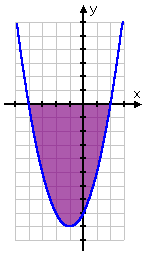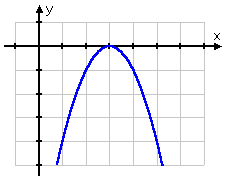Search

Solving Quadratic Inequalities: Examples (page 3 of 3)• Solve x2 + 2x  8 < 0.

First, I'll find the zeroes:

x2 + 2x  8 = 0
(x + 4)(x  2) = 0

x = 4
or  x = 2

These x-intercepts split the number line into three intervals: x < 4, 4 < x < 2, and x > 2. Since this is a "less than" inequality, I need the intervals where the parabola is below the x-axis. Since the graph of y = x2 + 2x  8 is a right-side-up parabola, it is below the axis in the middle (between the two intercepts). Since this is an "or equal to" inequality, the boundary points of the intervals (the intercepts themselves) are included in the solution.

Then the solution is: 4 < x < 2

 You can check the answer from the graph:There is one fiddly case that you might not even have to deal with, but I'll cover it anyway, just in case your teacher likes tricky test problems.

• Solve x2 + 6x  9 > 0.

First, I'll find the zeroes of y = x2 + 6x  9, the associated quadratic equation:

x2 + 6x  9 = 0
x2  6x + 9 = 0

(x  3)(x  3) = 0

x = 3

 In this case, there is exactly one x-intercept. When you have only one intercept like this, the quadratic doesn't cross the axis, but instead just touches it, as you can see here:Advertisement

I need to find where y = x2 + 6x  9 is above the axis. But I know (and can verify from the above graph) that this quadratic only touches the axis from below; it is never fully above the axis. However, this inequality is an "or equal to" inequality, so the "equal" part counts as part of the solution. That is, the intercept is part of the solution. In this case, it is actually the only solution, because the graph only touches the axis (is equal to zero); it never goes above (is never greater than zero).

So the solution is

x = 3

By the way, if you're supposed to write your solutions in set notation, a single-element set like this is written as "{3}" (those are curly-braces, not parentheses), and is called a "singleton set".

In contrast to the previous solution, look at this:

• Solve x2 + 6x  9 > 0.

In this case, they're asking me for where the graph is strictly above the axis. Since the graph only touches the axis from below, and never crosses, then the graph is never above the axis, so there is:   Copyright © Elizabeth Stapel 2002-2011 All Rights Reserved

no solution.

If you are careful about finding the zeroes of the quadratic, and use your knowledge of the shape of quadratic graphs, you shouldn't have any trouble solving quadratic inequalities.

<< Previous  Top  |  1 | 2 | 3  |  Return to Index

 Cite this article as: Stapel, Elizabeth. "Solving Quadratic Inequalities: Examples." Purplemath. Available from     https://www.purplemath.com/modules/ineqquad3.htm. Accessed [Date] [Month] 2016

Study Skills Survey

Tutoring from Purplemath
Find a local math tutor

 Copyright © 2021  Elizabeth Stapel   |   About   |   Terms of Use   |   Linking   |   Site Licensing Contact Us